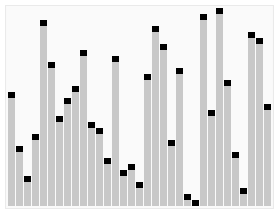# Ricardo Borges

Personal blog## Quick Sort

Quick Sort is an in-place sorting algorithm that uses a divide-and-conquer technique to sort a given list, which means it breaks down a problem into smaller parts in order to be simpler to solve and doesn't uses auxiliary data structures.

Quick Sort starts by selecting an element from the list to be the pivot, usually is the first or last element.

Next, rearrange the other elements, so all elements smaller than the pivot go to its left and all elements greater than the pivot goes to its right.

This process is repeated for the right and left sides of the pivot.## Implementation

Here is a recursive implementation of Quick Sort in TypeScript

``````1/**
2 * swap function
3 */
4export function swap(arr: number[], i: number, j: number) {
5  [arr[i], arr[j]] = [arr[j], arr[i]];
6}
7
8/**
9 * recursive function
10 */
11function quickSort(arr: number[], start: number, end: number) {
12  // base case
13  if (start >= end) return;
14
15  // rearrange the entire array based on a pivot
16  // this pointer is the middle of the array, it will be explained in the next function
17  const pointer = rearrange(arr, start, end);
18
19  // rearrange the left and right side of the pivot
20  quickSort(arr, start, pointer - 1);
21  quickSort(arr, pointer + 1, end);
22}
23
24/**
25 * function to rearrange the array based on a pivot
26 */
27function rearrange(arr: number[], start: number, end: number) {
28  // choose last element to be the pivot
29  const pivot = arr[end];
30
31  // this pointer works like a placeholder for the pivot, at the end, it will be in the middle of the array, and will swap with the pivot that is in the end
32  let pointer = start;
33
34  for (let i = start; i < end; i++) {
35    if (arr[i] < pivot) {
36      swap(arr, pointer, i);
37
38      // increment pointer, so it stays ahead of the elements that are smaller than the pivot
39      pointer++;
40    }
41  }
42
43  // put the pivot in the middle
44  swap(arr, pointer, end);
45  return pointer;
46}
47
48const arr = [3, 7, 2, 6, 5];
49quickSort(arr, 0, arr.length - 1);
50console.log(arr); // 2, 3, 5, 6, 7``````

The important part of Quick Sort is the choice of the pivot, since a bad choice leads to the worst-case time complexity, speaking of which, the average case time complexity of this algorithm is O(n log n), while in the worst case is O(n²), however, the worst-case can be avoided using a randomized version of this algorithm. Also, unlike Merge Sort, Quick Sort is an in-place algorithm, which gives it the advantage of having a constant space complexity O(1).